#### Chapter 4 Algebraic Identities R.D. Sharma Solutions for Class 9th Math MCQs

Multiple Choice Questions

1. Mark the correct alternative in each of the following:Solution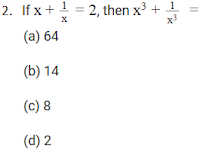Solution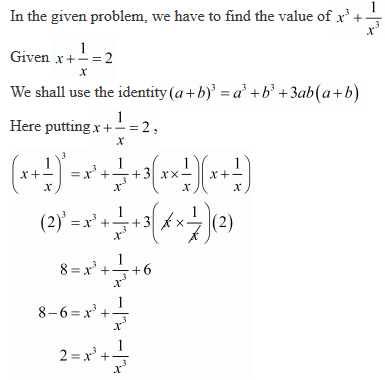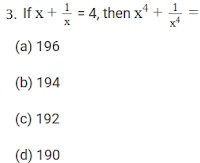SolutionSolutionSolutionSolutionSolution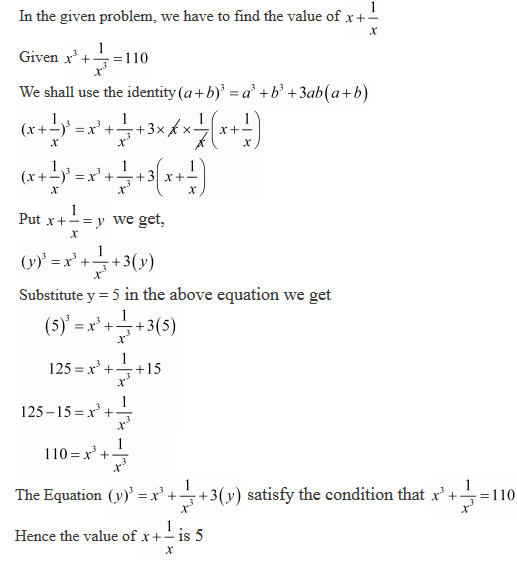SolutionSolution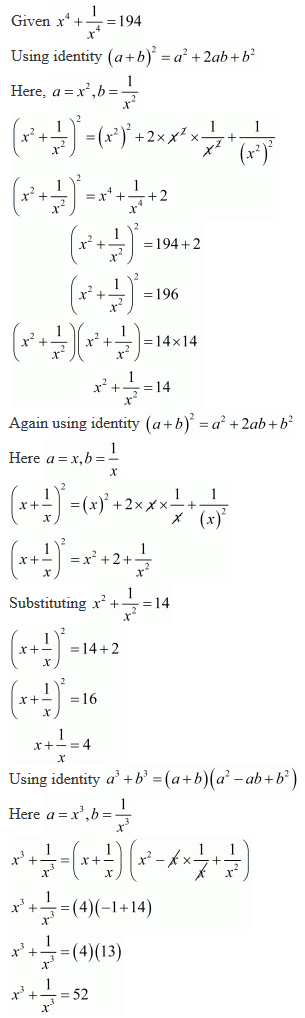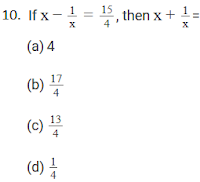Solution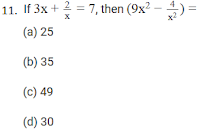Solution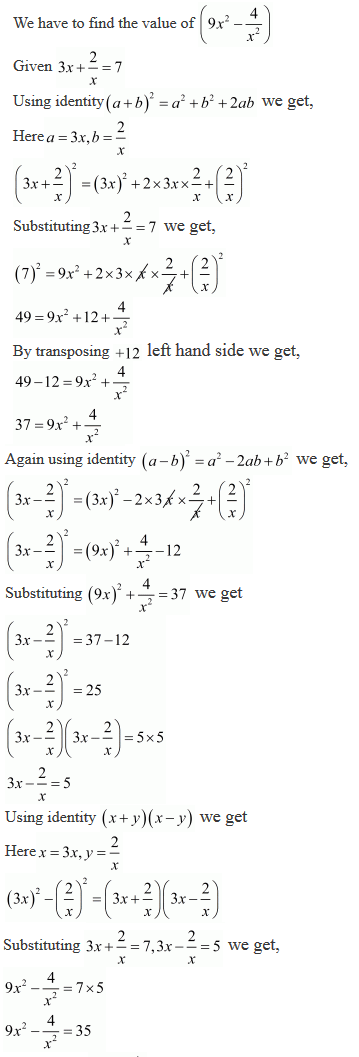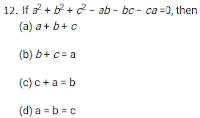Solution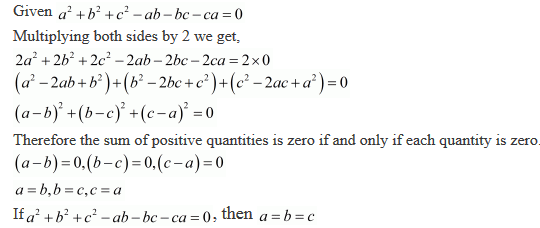Solution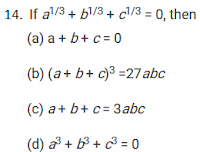Solution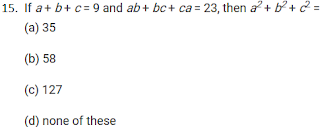Solution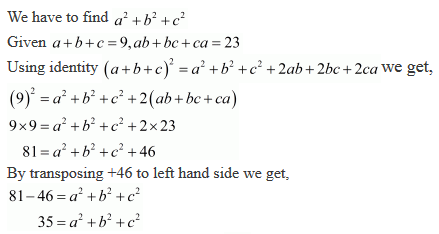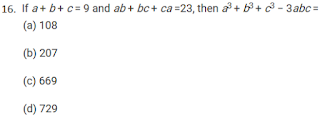Solution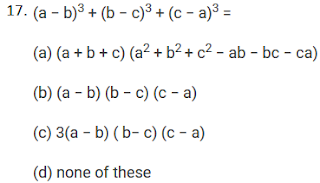SolutionSolution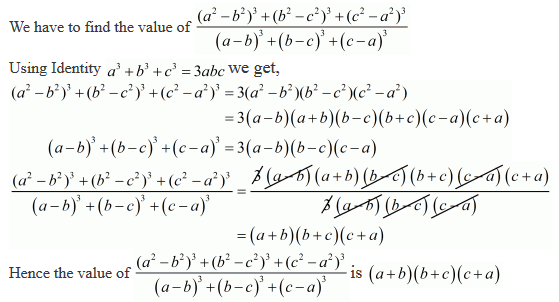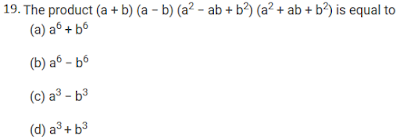SolutionSolution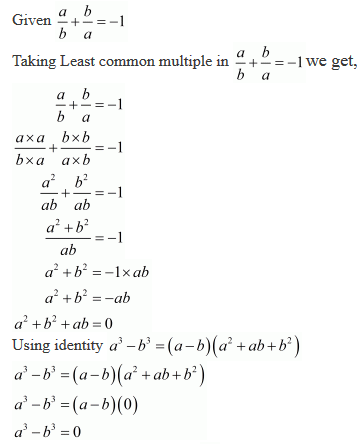Solution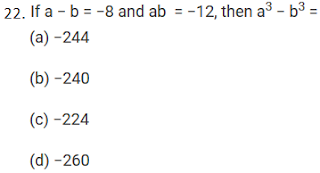Solution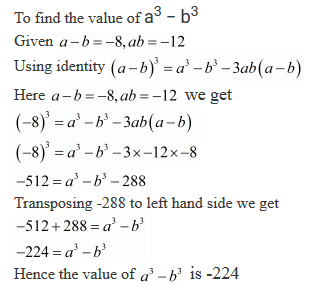SolutionSolution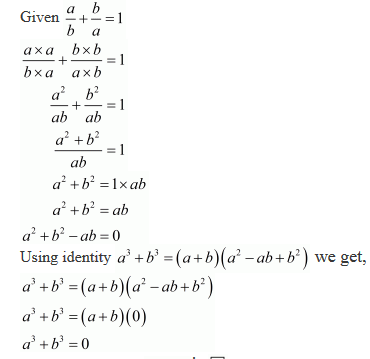25. 75 × 75 + 2 × 75 × 25 + 25 × 25 is equal to
(a) 10000
(b) 6250
(c) 7500
(d) 3750

Solution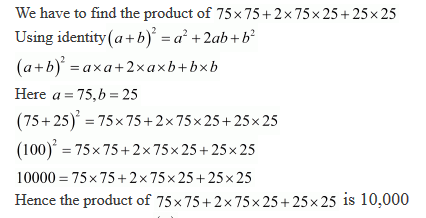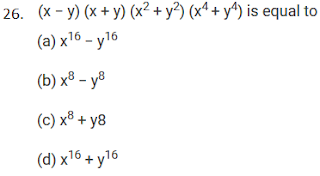Solution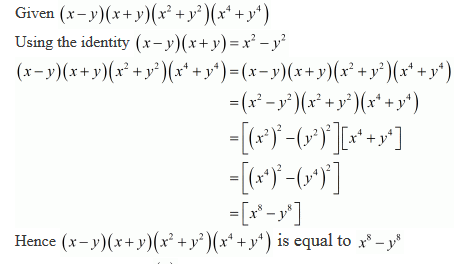Solution